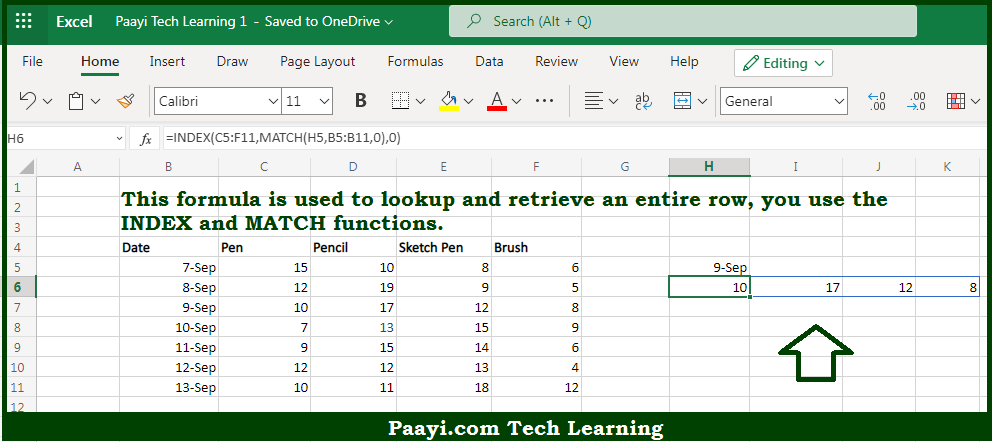# Learn How to Lookup Entire Row in Microsoft Excel

Written by | 0 Comments | 250 Views

In this article, you will learn how to evaluate things based on the LOOKUP function in Microsoft Excel using a single/combination(s) of functions. You will also know how to Lookup Entire Row and see the generic formula.

Learn How to Lookup Entire Row in Microsoft Excel

The main purpose of this formula is to lookup and retrieve an entire row. Here we will learn how to perform lookup for the entire row in the given data range in the workbook in Microsoft Excel. That implies, with the help of a formula based on the INDEX, and MATCH functions you can able to perform lookup and retrieve an entire row. So, with the help of this formula, you can able to perform lookup for an entire row in the given data range in the workbook in Microsoft Excel.

General Formula to Lookup Entire Row

The Explanation to Lookup Entire RowSo we know that with the help of the given formula above you can able lookup and retrieve an entire row. Here we will learn how to perform lookup for the entire row in the given data range in the workbook in Microsoft Excel. As we know the MATCH function is used to identify the row index, then INDEX retrieves the entire row by setting the row number to zero. Working from the inside out, MATCH is used to get the row index. So now you have learned how to perform the lookup for an entire row in the given data range in the workbook in Microsoft Excel.